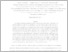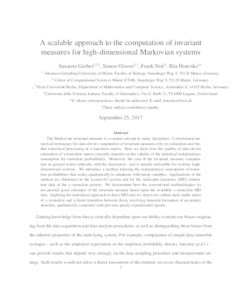Repository: Freie Universität Berlin, Math Department

# A scalable approach to the computation of invariant measures for high-dimensional Markovian systems

Gerber, S. and Olsson, S. and Noé, F. and Horenko, I. (2018) A scalable approach to the computation of invariant measures for high-dimensional Markovian systems. Sci. Rep., 8 (1796). ISSN 2045-2322Preview

1MB

Official URL: http://dx.doi.org/10.1038/s41598-018-19863-4

## Abstract

The Markovian invariant measure is a central concept in many disciplines. Conventional numerical techniques for data-driven computation of invariant measures rely on estimation and further numerical processing of a transition matrix. Here we show how the quality of data-driven estimation of a transition matrix crucially depends on the validity of the statistical independence assumption for transition probabilities. Moreover, the cost of the invariant measure computation in general scales cubically with the dimension - and is usually unfeasible for realistic highdimensional systems. We introduce a method relaxing the independence assumption of transition probabilities that scales quadratically in situations with latent variables. Applications of the method are illustrated on the Lorenz-63 system and for the molecular dynamics (MD) simulation data of the alpha-synuclein protein. We demonstrate how the conventional methodologies do not provide good estimates of the invariant measure based upon the available alpha-synuklein MD data. Applying the introduced approach to these MD data we detect two robust meta-stable states of alpha-synuclein and a linear transition between them, involving transient formation of secondary structure, qualitatively consistent with previous purely experimental reports.

Item Type: Article SFB 1114 Preprint 09/2017 Mathematical and Computer Sciences > Mathematics > Applied Mathematics Department of Mathematics and Computer Science > Institute of Mathematics > Geophysical Fluid Dynamics Group 2114 Silvia Hoemke 29 Sep 2017 11:41 20 Feb 2018 13:56

Repository Staff Only: item control page